# ISEE Upper Level Quantitative : How to subtract variables

## Example Questions

### Example Question #1 : How To Subtract Variables

Simplify: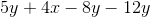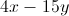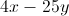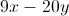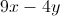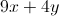Explanation: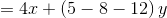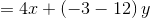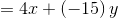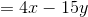### Example Question #2 : How To Subtract Variables

Simplify: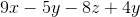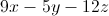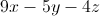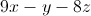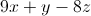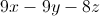Explanation: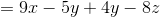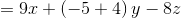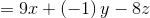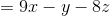### Example Question #3 : How To Subtract Variablesis negative. Which of these quantities is the greater?

(A)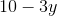(B)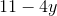(A) is greater

It is impossible to determine which is greater from the information given

(B) is greater

(A) and (B) are equal

(B) is greater

Explanation: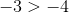,

So by the multiplication property of inequality, when each is multiplied by the negative number,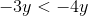.

Also,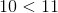,

so by the addition property of inequality,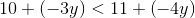or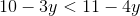This makes (B) greater.

### Example Question #1 : How To Subtract Variables

Assume you know the values of all four variables in the expression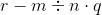In which order do you perform the operations in order to evaluate the expression?

Multiply, divide, subtract

Subtract, divide, multiply

Subtract, multiply, divide

Multiply, divide, subtract

Divide, multiply, subtract

Divide, multiply, subtract

Explanation:

Multiplication and division take precedence over subtraction in the order of operations, so these two operations are performed first. The two must be performed from left to right, so the division is worked first, followed by the multiplication. The subtraction is last.

### Example Question #2 : How To Subtract Variables

Consider the expression: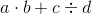Which of the following expressions must be equal in value to the above expression?

I)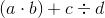II)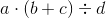III)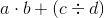I and II only

III only

I and III only

I, II, and III

I only

I and III only

Explanation:

The order of operations is as follows:

Exponents

Multiplication and division (left to right)

Addition and subtraction (left to right)

The expressionis therefore evaluated by multiplying, then dividing, then adding. The net result is that the product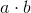is added to the quotient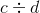.

If we examine (I), we see that, since the multiplication is in parentheses, it is worked first. The division is worked second, then the addition. The order of operations has not changed, so the expressions are equivalent.

If we examine (II), we see that the order of operations has changed so that the addition is worked first. We see through example that the expressions can have different values: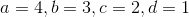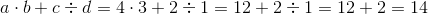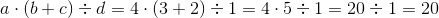If we examine (III), we see that, since the division is in parentheses, it is worked first. The multiplication is worked second, then the addition. The upshot is the same as in the main expression, however - the productis added to the quotient. Therefore, the expressions are equivalent.

The correct response is (I) and (III)

### Example Question #2 : How To Subtract Variables

When evaluating the expression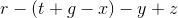,

assuming you know the values of all five variables, what is the third operation that must be performed?

The leftmost subtraction

The rightmost subtraction